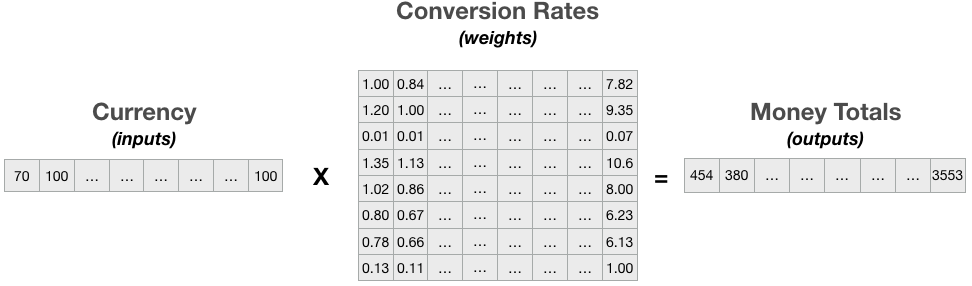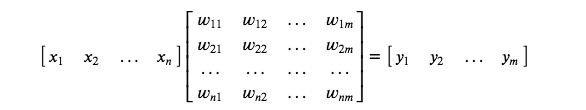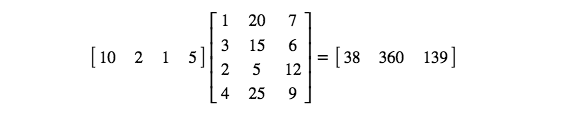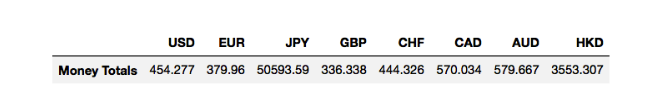# Python:线性代数-矩阵乘法实战 Lab (五十三)

## 货币换算问题### 编写货币换算问题代码

import numpy as np
import pandas as pd

# Creates numpy vector from a list to represent money (inputs) vector.
money = np.asarray([70, 100, 20, 80, 40, 70, 60, 100])

# Creates pandas dataframe with column labels(currency_label) from the numpy vector for printing.
currency_label = ["USD", "EUR", "JPY", "GBP", "CHF", "CAD", "AUD", "HKD"]
money_df = pd.DataFrame(data=money, index=currency_label, columns=["Amounts"])
print("Inputs Vector:")
money_df.T

Inputs Vector:
USD EUR JPY GBP CHF CAD AUD HKD
Amounts 70 100 20 80 40 70 60 100

# Sets path variable to the 'path' of the CSV file that contains the conversion rates(weights) matrix.
path = %pwd

# Imports conversion rates(weights) matrix as a pandas dataframe.

# Creates numpy matrix from a pandas dataframe to create the conversion rates(weights) matrix.
conversion_rates = conversion_rates_df.values

# Prints conversion rates matrix.
print("Weights Matrix:")
conversion_rates_df

Weights Matrix:
USD EUR JPY GBP CHF CAD AUD HKD
USD-To 1.0000 0.8364 111.3700 0.7404 0.9781 1.2548 1.2760 7.8218
EUR-To 1.1956 1.0000 133.1540 0.8852 1.1694 1.5002 1.5256 9.3517
JPY-To 0.0090 0.0075 1.0000 0.0066 0.0088 0.0113 0.0115 0.0702
GBP-To 1.3507 1.1297 150.4275 1.0000 1.3211 1.6949 1.7235 10.5649
CHF-To 1.0224 0.8551 113.8636 0.7569 1.0000 1.2829 1.3046 7.9969
CAD-To 0.7969 0.6666 88.7552 0.5900 0.7795 1.0000 1.0169 6.2335
AUD-To 0.7837 0.6555 87.2807 0.5802 0.7665 0.9834 1.0000 6.1299
HKD-To 0.1278 0.1069 14.2384 0.0947 0.1250 0.1604 0.1631 1.0000## TODO：矩阵乘法

# TODO 1.: Calculates the money totals(outputs) vector using matrix multiplication in numpy.
money_totals = np.matmul(money, conversion_rates)

# Converts the resulting money totals vector into a dataframe for printing.
money_totals_df = pd.DataFrame(data = money_totals, index = currency_label, columns = ["Money Totals"])
print("Outputs Vector:")
money_totals_df.T

Outputs Vector:
USD EUR JPY GBP CHF CAD AUD HKD
Money Totals 454.277 379.96 50593.59 336.338 444.326 570.034 579.667 3553.307

### 通过矩阵乘法进行货币换算的解决方案